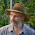## 16 December 2016

### Albrecht Dürer's amazing magic square

This "magic square" was incorporated by Dürer in his engraving "Melencolia I" (full image below)
In recreational mathematics, a magic square is a n × n square grid - where n is the number of rows (or columns) - filled with distinct positive integers in the range of [1.. n2] such that the sum of the integers in each row, column or diagonal equals a same value...

The order-4 magic square Albrecht Dürer immortalized in his 1514 engraving Melencolia I, referred to above, is believed to be the first seen in European art. It is very similar to Yang Hui's square, which was created in China about 250 years before Dürer's time. The sum 34 can be found in the rows, columns, diagonals, each of the quadrants, the center four squares, and the corner squares (of the 4×4 as well as the four contained 3×3 grids). This sum can also be found in the four outer numbers clockwise from the corners (3+8+14+9) and likewise the four counter-clockwise (the locations of four queens in the two solutions of the 4 queens puzzle), the two sets of four symmetrical numbers (2+8+9+15 and 3+5+12+14), the sum of the middle two entries of the two outer columns and rows (5+9+8+12 and 3+2+15+14), and in four kite or cross shaped quartets (3+5+11+15, 2+10+8+14, 3+9+7+15, and 2+6+12+14). The two numbers in the middle of the bottom row give the date of the engraving: 1514. The numbers 1 and 4 at either side of the date correspond respectively to the letters "A" and "D," which are the initials of the artist.
Very cool.

1.:-) And that is why the angel is so melancholic: "I'll never look at a four-square the same way again.". :-)

1.2.it makes you wonder how many other things are hidden in there.

I-)

3.This secret message, for one. 0110111...SORSOERIODT011011011011011011 obviously means "Five Centuryes hence [...] an Orange Monſtre ſhall Rise, & inundate ye Worlde in Much Grueſomeneſse"

4.that is the 'milk chocolate orange ball' that the prophecy speaks of. while the concept was well known back then, it took all that time to come up with the technology, and ingredients, to make them.

I-)

5.2.Well thanks, that brought me back 50ish years to reading Martin Gardner's More Mathematical Puzzles and Diversions [available for \$0.01 on Amazon!] until the thing fell apart. Chapter 12 Magic Squares features the Durer print, and Gardner has a couple of pages detailing what it all means and doesn't. Bri'nt!